To prove one-one & onto (injective, surjective, bijective)

Chapter 1 Class 12 Relation and Functions
Concept wise

f: X → Y

Function f is one-one if every element has a unique image,

i.e.

when f(x 1 ) = f(x 2 )

⇒ x 1 = x 2

Otherwise the function is many-one.

## How to check if function is one-one - Method 1

In this method, we check for each and every element manually if it has unique image

#### Check whether the following are one-one ?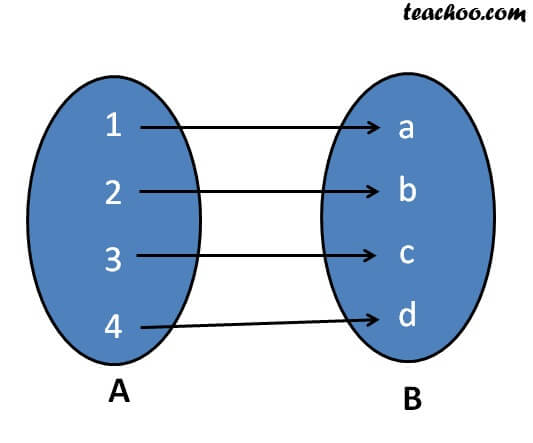Element Image 1 a 2 b 3 c 4 d

Since every element has a unique image,

it is one-one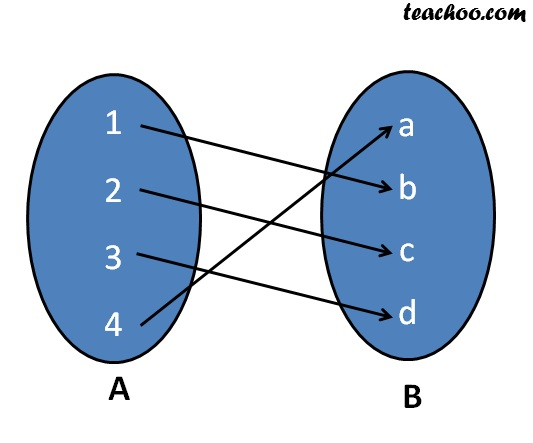Element Image 1 b 2 c 3 d 4 a

Since every element has a unique image,

it is one-one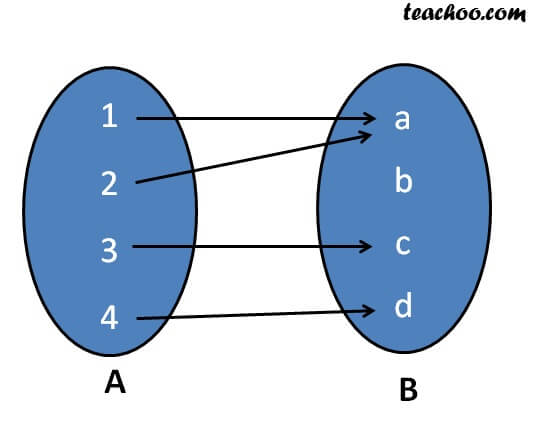Element Image 1 a 2 a 3 c 4 d

Since 1 and 2 has same image,

it is not one-one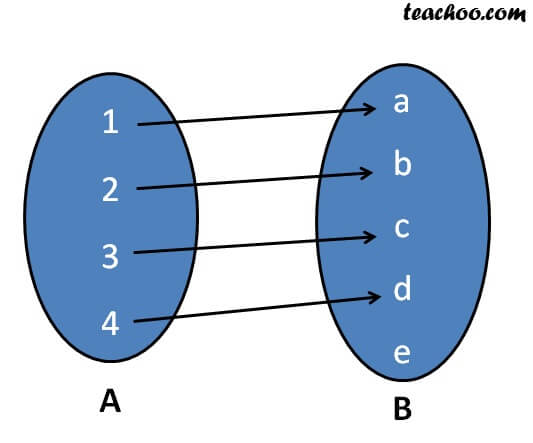Element Image 1 a 2 b 3 c 4 d

Since every element has a unique image,

it is one-one

## How to check if function is one-one -  Method 2

This method is used if there are large numbers

Example:

f : N N    (There are infinite number of natural numbers)

f : R R    (There are infinite number of real numbers)

f : Z Z     (There are infinite number of integers)

Steps :

How to check one-one?

1. Calculate f(x 1 )
2. Calculate f(x 2 )
3. Put f(x 1 ) = f(x 2 )

If x 1 = x 2 , then it is one-one.

Otherwise, many-one

Let’s take some examples

f: R R

f(x) = x

Is f one-one?

-a-

1. Calculate f(x 1 )
2. Calculate f(x 2 )
3. Put f(x 1 ) = f(x 2 ),
4. If x 1 = x 2 , then it is one-one.
Otherwise, many-one

f(x 1 ) = x 1

f(x 2 ) = x 2

Putting f(x 1 ) = f(x 2 )

x 1 = x 2

Since x 1 = x 2 ,

f is one-one.

-ea-

f: R R

f(x) = 1

Is f one-one?

-a-

Since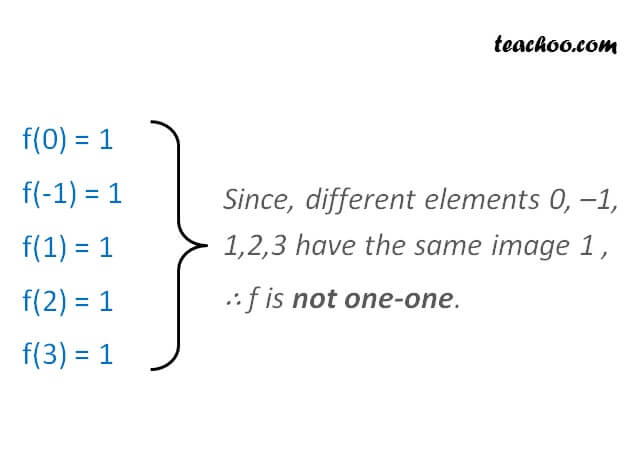f is not one-one.

-ea-

Learn in your speed, with individual attention - Teachoo Maths 1-on-1 Class